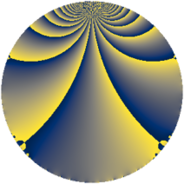# Properties

 Label 325.2.oLevel $325$ Weight $2$ Character orbit 325.o Rep. character $\chi_{325}(74,\cdot)$ Character field $\Q(\zeta_{6})$ Dimension $36$ Newform subspaces $3$ Sturm bound $70$ Trace bound $4$

# Related objects

## Defining parameters

 Level: $$N$$ $$=$$ $$325 = 5^{2} \cdot 13$$ Weight: $$k$$ $$=$$ $$2$$ Character orbit: $$[\chi]$$ $$=$$ 325.o (of order $$6$$ and degree $$2$$) Character conductor: $$\operatorname{cond}(\chi)$$ $$=$$ $$65$$ Character field: $$\Q(\zeta_{6})$$ Newform subspaces: $$3$$ Sturm bound: $$70$$ Trace bound: $$4$$ Distinguishing $$T_p$$: $$2$$

## Dimensions

The following table gives the dimensions of various subspaces of $$M_{2}(325, [\chi])$$.

Total New Old
Modular forms 84 44 40
Cusp forms 60 36 24
Eisenstein series 24 8 16

## Trace form

 $$36q + 16q^{4} + 6q^{6} + 8q^{9} + O(q^{10})$$ $$36q + 16q^{4} + 6q^{6} + 8q^{9} - 6q^{11} + 40q^{14} + 4q^{16} - 8q^{19} - 20q^{24} - 30q^{26} - 10q^{29} - 32q^{31} - 84q^{34} + 42q^{36} + 20q^{39} - 36q^{41} - 8q^{44} - 14q^{46} - 2q^{49} - 48q^{51} + 58q^{54} + 2q^{56} - 10q^{61} + 20q^{64} + 68q^{66} + 8q^{69} + 2q^{71} - 16q^{74} + 4q^{76} + 72q^{79} - 18q^{81} - 104q^{84} - 48q^{86} - 52q^{89} - 6q^{91} - 70q^{94} + 76q^{96} - 176q^{99} + O(q^{100})$$

## Decomposition of $$S_{2}^{\mathrm{new}}(325, [\chi])$$ into newform subspaces

Label Dim. $$A$$ Field CM Traces $q$-expansion
$$a_2$$ $$a_3$$ $$a_5$$ $$a_7$$
325.2.o.a $$8$$ $$2.595$$ 8.0.12960000.1 None $$0$$ $$0$$ $$0$$ $$0$$ $$q+\beta _{6}q^{2}+(\beta _{3}+2\beta _{6}+\beta _{7})q^{3}+(-\beta _{2}+\cdots)q^{4}+\cdots$$
325.2.o.b $$8$$ $$2.595$$ 8.0.592240896.1 None $$0$$ $$0$$ $$0$$ $$0$$ $$q+\beta _{1}q^{2}+\beta _{2}q^{3}+(1-2\beta _{3}+\beta _{4}+\beta _{6}+\cdots)q^{4}+\cdots$$
325.2.o.c $$20$$ $$2.595$$ $$\mathbb{Q}[x]/(x^{20} - \cdots)$$ None $$0$$ $$0$$ $$0$$ $$0$$ $$q+\beta _{1}q^{2}-\beta _{10}q^{3}+(\beta _{7}+\beta _{11})q^{4}+\cdots$$

## Decomposition of $$S_{2}^{\mathrm{old}}(325, [\chi])$$ into lower level spaces

$$S_{2}^{\mathrm{old}}(325, [\chi]) \cong$$ $$S_{2}^{\mathrm{new}}(65, [\chi])$$$$^{\oplus 2}$$